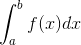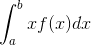Open in App
Not now

# ISRO | ISRO CS 2009 | Question 76

• Last Updated : 01 Jun, 2018

Let f(x) be the continuous probability density function of a random variable x, the probability that a < x ≤ b, is (A) f (b − a)
(B) f (b) − f (a)
(C)(D)